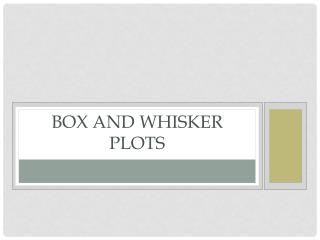DownloadDownload PresentationBox and whisker plots

# Box and whisker plots

Télécharger la présentation## Box and whisker plots

- - - - - - - - - - - - - - - - - - - - - - - - - - - E N D - - - - - - - - - - - - - - - - - - - - - - - - - - -
##### Presentation Transcript

1. Box and whisker plots

2. What is a box and whisker plot?

3. Your task • When I say the magic words, you will silently stand and make a line at the front of the room from shortest to tallest. NO TALKING ALLOWED! • If someone is the same height as you, you need to stand next to that student. • Make sure you are all shoulder to shoulder.

4. Median • Who is the median? Hold up the median card. • We now have two halves. • 1 half is the taller group • 1 half is the shorter group

5. What is a quartile? • The quartiles of the data set are the middle of each half. • Who is in the middle of the shorter half? This is the lower quartile. Hold up the lower quartile card. • Who is in the middle of the taller half? This is the upper quartile. Hold up the upper quartile card.

6. What are endpoints? • The max and minimum of your data. • In this case, the shortest students and the tallest student are both the endpoints. • The shortest student is the lower endpoint and the tallest in the upper endpoint.

7. All students except for the median, upper and lower quartiles, and upper and lower endpoints may return to their seats.

8. Box and whisker plots • The BOX runs from the lower quartile to upper quartile. • The median is always located inside the BOX (space from lower quartile to upper quartile.) • The median does not have to be in the middle of the box.

9. WHISKERS • The WHISKERS run from the lower quartile to the endpoint and from the upper quartile to the endpoint.

10. How to create a box and whisker on paper • Organize your data from least to greatest • Make a number line that begins a bit before and extends a bit after your endpoint. For example, if your endpoints are 131 and 182, I would have my number line range from 130 to 185. • Next, plot the five points on the number line (median, lower quartile, upper quartile, lower endpoint, upper endpoint.) • Draw the box connecting the two quartiles (upper and lower.) • Draw a straight line through the box to show the median. • Last draw in the whiskers, quartile to endpoints.

11. Let’s Try it TOGETHER!!! • Use this set of data to create a Box and Whiskers Plot:

12. WARM-UP • On a piece of lined paper, please use the data below to create a Box and Whiskers Plot: